# 591 and a Sometimes Impossible Level 6

5 + 9 + 1 = 15, a multiple of 3 so 591 is divisible by 3.

However, since 9 is a multiple of 3, it isn’t necessary to include it in the equation, so I simple say 5 + 1 = 6, a multiple of 3 so 591 is divisible by 3.

591 is the hypotenuse of the Pythagorean triple 84-585-591. Which of 591’s factors is the greatest common factor of those three numbers?

—————————————————————————————————

I love that Joseph Nebus wrote that the FIND THE FACTORS puzzles are “a fun recreational mathematics puzzle even if the level 6’s and sometimes level 5’s will sometimes feel impossible.”

Judge for yourself. Is this Level 6 puzzle impossible or not!Print the puzzles or type the solution on this excel file: 10 Factors 2015-08-17

—————————————————————————————————

• 591 is a composite number.
• Prime factorization: 591 = 3 x 197
• The exponents in the prime factorization are 1 and 1. Adding one to each and multiplying we get (1 + 1)(1 + 1) = 2 x 2 = 4. Therefore 591 has exactly 4 factors.
• Factors of 591: 1, 3, 197, 591
• Factor pairs: 591 = 1 x 591 or 3 x 197
• 591 has no square factors that allow its square root to be simplified. √591 ≈ 24.31049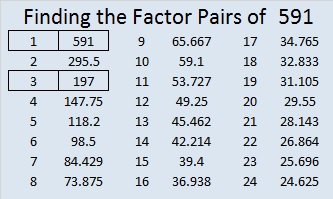—————————————————————————————————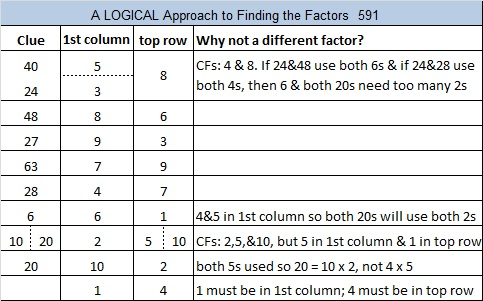# 590 and Level 5

3(20^2)/2 – 20/2 = 600 – 10 = 590. That means that 590 is the 20th pentagonal number.

590 is also the hypotenuse of the Pythagorean triple 354-472-590. Which factor of 590 is the greatest common factor of those three numbers? Hint: In this case the GCF is the difference between the middle number in the triple and either of the other two numbers.Print the puzzles or type the solution on this excel file: 10 Factors 2015-08-17

—————————————————————————————————

• 590 is a composite number.
• Prime factorization: 590 = 2 x 5 x 59
• The exponents in the prime factorization are 1, 1, and 1. Adding one to each and multiplying we get (1 + 1)(1 + 1)(1 + 1) = 2 x 2 x 2 = 8. Therefore 590 has exactly 8 factors.
• Factors of 590: 1, 2, 5, 10, 59, 118, 295, 590
• Factor pairs: 590 = 1 x 590, 2 x 295, 5 x 118, or 10 x 59
• 590 has no square factors that allow its square root to be simplified. √590 ≈ 24.2899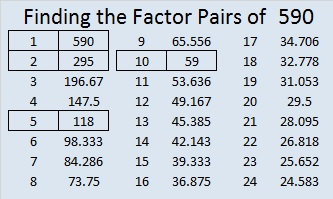—————————————————————————————————# 586 and Level 1

586 is the hypotenuse of the Pythagorean triple 136-570-586. Which factor of 586 is the greatest common factor of those three numbers?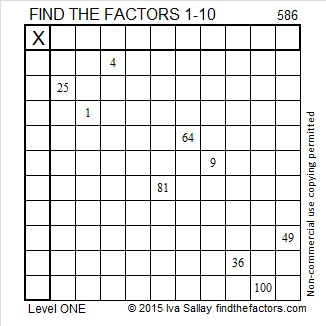Print the puzzles or type the solution on this excel file: 10 Factors 2015-08-17

—————————————————————————————————

• 586 is a composite number.
• Prime factorization: 586 = 2 x 293
• The exponents in the prime factorization are 1 and 1. Adding one to each and multiplying we get (1 + 1)(1 + 1) = 2 x 2 = 4. Therefore 586 has exactly 4 factors.
• Factors of 586: 1, 2, 293, 586
• Factor pairs: 586 = 1 x 586 or 2 x 293
• 586 has no square factors that allow its square root to be simplified. √586 ≈ 24.2074—————————————————————————————————# 583 The secret to solving these puzzles is using logic and never guessing

5 – 8 + 3 = 0. Since zero is divisible by 11 we know that 583 is also divisible by 11.

583 is the sum of all the prime numbers from 31 to 73. Add them up and see for yourself.

583 is also the hypotenuse of the Pythagorean triple 308-495-583. What is the greatest common factor of those three numbers?

—————————————————————————————————

The secret to solving these puzzles is using logic and never guessing. That means NEVER write a factor in a cell unless you know for sure it belongs there. Not following this advice can cause frustration when the puzzles are only intended to be fun. Read How to Solve a FIND THE FACTORS puzzle for more instructions.

###—————————————————————————————————

• 583 is a composite number.
• Prime factorization: 583 = 11 x 53
• The exponents in the prime factorization are 1 and 1. Adding one to each and multiplying we get (1 + 1)(1 + 1) = 2 x 2 = 4. Therefore 583 has exactly 4 factors.
• Factors of 583: 1, 11, 53, 583
• Factor pairs: 583 = 1 x 583 or 11 x 53
• 583 has no square factors that allow its square root to be simplified. √583 ≈ 24.14539—————————————————————————————————# 582 and Level 4

582 is the sum of all the prime numbers from 59 to 89. Can you list those eight consecutive primes?

582 is also the hypotenuse of the Pythagorean triple 390-432-582. Which of 582’s factors is the greatest common factor of those three numbers?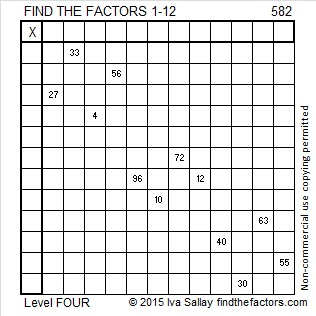Print the puzzles or type the solution on this excel file: 12 Factors 2015-08-10

—————————————————————————————————

• 582 is a composite number.
• Prime factorization: 582 = 2 x 3 x 97
• The exponents in the prime factorization are 1, 1, and 1. Adding one to each and multiplying we get (1 + 1)(1 + 1)(1 + 1) = 2 x 2 x 2 = 8. Therefore 582 has exactly 8 factors.
• Factors of 582: 1, 2, 3, 6, 97, 194, 291, 582
• Factor pairs: 582 = 1 x 582, 2 x 291, 3 x 194, or 6 x 97
• 582 has no square factors that allow its square root to be simplified. √582 ≈ 24.124676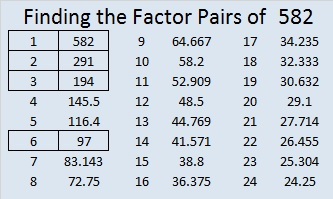—————————————————————————————————# 579 and Level 1

579 is the hypotenuse of the Pythagorean triple 285-504-579. Which of 579’s factors is the greatest common factor of those three numbers?

Last week someone googled “find,the least 6 digit which has 173 factor” and arrived at Findthefactors.com. Here how to find the answer: Divide 100,000 by 173 and get 578.03 approximately. Round that answer up to 579. Multiply 579 by 173 and get 100167, the smallest 6-digit number that has 173 as a factor.Print the puzzles or type the solution on this excel file: 12 Factors 2015-08-10

—————————————————————————————————

• 579 is a composite number.
• Prime factorization: 579 = 3 x 193
• The exponents in the prime factorization are 1 and 1. Adding one to each and multiplying we get (1 + 1)(1 + 1) = 2 x 2 = 4. Therefore 579 has exactly 4 factors.
• Factors of 579: 1, 3, 193, 579
• Factor pairs: 579 = 1 x 579 or 3 x 193
• 579 has no square factors that allow its square root to be simplified. √579 ≈ 24.0624188—————————————————————————————————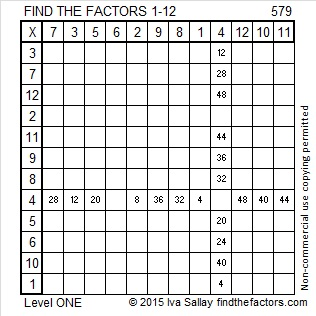# 574 Start at the top of the first column and fly down one cell at a time to solve this Level 3 puzzle

One of 574’s factors is the hypotenuse of a primitive Pythagorean triple so 574 is the hypotenuse of the triple 126-560-574. Which of 574’s factors is the greatest common factor of those three numbers?Print the puzzles or type the solution on this excel file: 10 Factors 2015-08-03

—————————————————————————————————

• 574 is a composite number.
• Prime factorization: 574 = 2 x 7 x 41
• The exponents in the prime factorization are 1, 1, and 1. Adding one to each and multiplying we get (1 + 1)(1 + 1)(1 + 1) = 2 x 2 x 2 = 8. Therefore 574 has exactly 8 factors.
• Factors of 574: 1, 2, 7, 14, 41, 82, 287, 574
• Factor pairs: 574 = 1 x 574, 2 x 287, 7 x 82, or 14 x 41
• 574 has no square factors that allow its square root to be simplified. √574 ≈ 23.958297.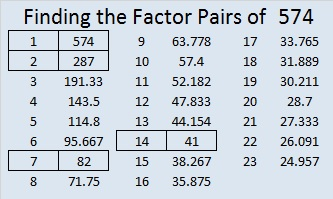—————————————————————————————————

A Logical Approach to solve a FIND THE FACTORS puzzle: Find the column or row with two clues and find their common factor. Write the corresponding factors in the factor column (1st column) and factor row (top row).  Because this is a level three puzzle, you have now written a factor at the top of the factor column. Continue to work from the top of the factor column to the bottom, finding factors and filling in the factor column and the factor row one cell at a time as you go.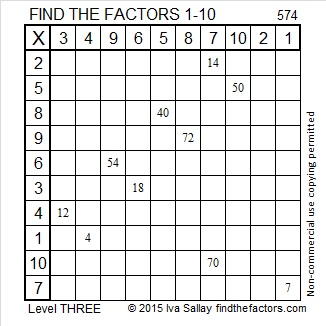# 572 and Level 1

72 can be evenly divided by 4 so 572 is divisible by 4.

5 – 7 + 2 = 0, which can be evenly divided by 11, so 11 is a factor of 572.

572 is the hypotenuse of the Pythagorean triple 220-528-572. Which factor of 572 is the greatest common factor of those three numbers?Print the puzzles or type the solution on this excel file: 10 Factors 2015-08-03

—————————————————————————————————

• 572 is a composite number.
• Prime factorization: 572 = 2 x 2 x 11 x 13, which can be written 572 = (2^2) x 11 x 13
• The exponents in the prime factorization are 2, 1, and 1. Adding one to each and multiplying we get (2 + 1)(1 + 1)(1 + 1) = 3 x 2 x 2 = 12. Therefore 572 has exactly 12 factors.
• Factors of 572: 1, 2, 4, 11, 13, 22, 26, 44, 52, 143, 286, 572
• Factor pairs: 572 = 1 x 572, 2 x 286, 4 x 143, 11 x 52, 13 x 44, or 22 x 26
• Taking the factor pair with the largest square number factor, we get √572 = (√4)(√143) = 2√143 ≈ 23.91652—————————————————————————————————# 565 and Level 1

565 is the sum of consecutive primes: 181 + 191 + 193 = 565.

565 is the sum of two squares two different ways: 565 = (22^2) + (9^2), and 565 = (23^2) + (6^2).

565 is the hypotenuse of four Pythagorean triples. The greatest common factor of two of them is 1 because they are primitives. Which of these triples are not primitive, and what is the greatest common factor of each of them?

• 75-560-565
• 276-493-565
• 339-452-565
• 396-403-565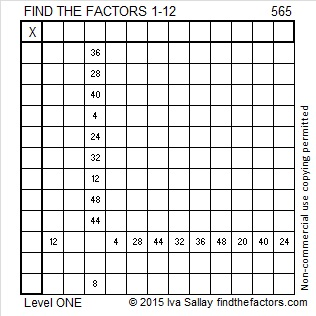Print the puzzles or type the solution on this excel file: 12 Factors 2015-07-27

—————————————————————————————————

• 565 is a composite number.
• Prime factorization: 565 = 5 x 113
• The exponents in the prime factorization are 1 and 1. Adding one to each and multiplying we get (1 + 1)(1 + 1) = 2 x 2 = 4. Therefore 565 has exactly 4 factors.
• Factors of 565: 1, 5, 113, 565
• Factor pairs: 565 = 1 x 565 or 5 x 113
• 565 has no square factors that allow its square root to be simplified. √565 ≈ 23.7697—————————————————————————————————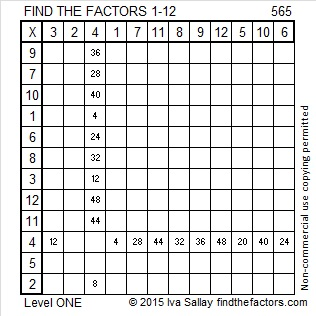# 562 and Level 4

562 is the sum of all the prime numbers from 23 to 71.

562 is also the hypotenuse of the Pythagorean triple 320-462-562. Which factor of 562 is the greatest common factor of those three numbers?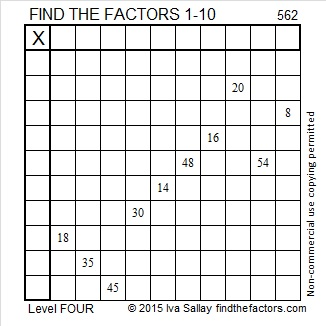Print the puzzles or type the solution on this excel file: 10 Factors 2015-07-20

—————————————————————————————————

• 562 is a composite number.
• Prime factorization: 562 = 2 x 281
• The exponents in the prime factorization are 1 and 1. Adding one to each and multiplying we get (1 + 1)(1 + 1) = 2 x 2 = 4. Therefore 562 has exactly 4 factors.
• Factors of 562: 1, 2, 281, 562
• Factor pairs: 562 = 1 x 562 or 2 x 281
• 562 has no square factors that allow its square root to be simplified. √562 ≈ 23.7065—————————————————————————————————